Latest Banking jobs   »   Quantitative Aptitude Quiz For SBI/IBPS PO...

Quantitative Aptitude Quiz For SBI/IBPS PO Mains 2021- 30th December

Directions (1-4): The following questions are accompanied by two statements A and B. You have to determine which statements(s) is/are sufficient/necessary to answer the questions.
(a) Statement ‘A’ alone is sufficient to answer the question, but statement ‘B’ alone is not sufficient to answer the questions.
(b) Statement ‘B’ alone is sufficient to answer the question, but statement ‘A’ alone is not sufficient to answer the question.
(c) Both the statements taken together are necessary to answer the questions, but neither of the statements alone is sufficient to answer the question.
(d) Either statement ‘A’ or statement ‘B’ by itself is sufficient to answer the question.
(e) Statements ‘A’ and ‘B’ taken together are not sufficient to answer the question.

Q1. Is ‘n’ even or odd integer?
(A) 3ⁿ – 1 is divisible by 4
(B) 5ⁿ – 1 is divisible by 4.

Q2. Is Sakshi earn profit or loss after selling a pen?
(A) Ratio of cost price of pen to mark price of pen is 1 : 3 and she gave Rs. 90 discount at the time of selling.
(B) If markup price of pen is decreased by 50% and Sakshi gave 50% discount on new mark price, then she losses Rs. 45.

Q3. Find the curved surface area of cylinder? [Radius of cylinder = 8 cm]
(A) Ratio between curved surface area of cylinder to lateral surface area of cone is 6 : 5 while radius and height of cylinder is same as radius and height of cone respectively.
(B) Total surface area of cylinder is 133 ⅓% more than curved surface area of cylinder.

Q4. Find the value of ‘R’?
(A) A man invested Rs. 2750 in the ratio of 5 : 6 at the rate of R% and 5% for four years on simple interest and get interest in the ratio of 5 : 4 respectively.
(B) Amir invested Rs. 500 & Rs. 800 at the rate of R% & (R + 2.5)% for two years respectively on simple interest. Amir get total Rs. 170 as interest.

Q5. A mixture contains ‘X’ litre milk and ‘Y’ litre water. If 30 litre of mixture is taken out and replaced with water such that ratio of milk and water becomes 1 : 1. But if 60 litre of mixture is taken out and replaced with water then ratio of milk and water becomes 1 : 2.
Quantity I: Value of ‘X’
Quantity II: Value of ‘Y + 40’
(a) Quantity I > Quantity II
(b) Quantity I < Quantity II
(c) Quantity I ≥ Quantity II
(d) Quantity I ≤ Quantity II
(e) Quantity I = Quantity II or No relation

Q6. There are three types of colored balls in a box. Probability of selecting one ball of ‘Blue color’ and one ball of red color is ⅖ and ⅓respectively. Number of blue color balls is 6 more than that of black color balls in the box.
Quantity I: Probability of selecting two black color balls from the box.
Quantity II: 1/18
(a) Quantity I > Quantity II
(b) Quantity I < Quantity II
(c) Quantity I ≥ Quantity II
(d) Quantity I ≤ Quantity II
(e) Quantity I = Quantity II or No relation

Q7. Quantity I : Days after which A and B meet. A and B set out to meet each other from two places 165 km apart. A travels 15 km the first day, 14 km second day, 13 km the third day and so on, B travels 10 km the first, 12 km the second day, 14 km the third day and so on.
Quantity II: Number of days required to complete the whole work if A, B and C can complete a piece of work in 10, 12 and 15 days respectively. A left the work 5 days before the work was completed and B left 2 days after A had left.
(a) Quantity I > Quantity II
(b) Quantity I < Quantity II
(c) Quantity I ≥ Quantity II
(d) Quantity I ≤ Quantity II
(e) Quantity I = Quantity II or No relation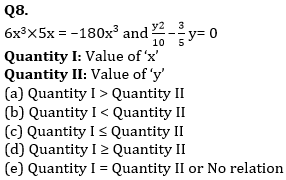Q9. Quantity I – Breadth of rectangle.
Sum of circumference of a circle and perimeter of a rectangle is 154 cm. and area of the circle is 346.5 cm². Length of the rectangle is 166 ⅔% more than radius of circle.
Quantity II – Side of square.
Circumference of a circle is 132 cm and area of circle is 1130 cm² more than area of square.
(a) Quantity I > Quantity II
(b) Quantity I < Quantity II
(c) Quantity I ≥ Quantity II
(d) Quantity I ≤ Quantity II
(e) Quantity I = Quantity II or no relation

Q10. Quantity I – Three times of amount invested by Gopal.
Arun and Gopal entered into a business with the capital of Rs. (P + 1200) and Rs. (P + 1500) respectively. After 8 months from starting, Arun withdrew half of his investment and Gopal doubled his investment. At the end of year Arun got Rs. 4250 out of total profit of Rs. 11250.
Quantity II – Profit share of C.
A and B started a business with initial investment of Rs. 27000 and Rs. 36000 respectively. After 4 months A withdraw Rs. 5000, B added Rs. 6000 and C joined with Rs. 35000. At the end of an year total profit of Rs. 130500 is obtained.
(a) Quantity I > Quantity II
(b) Quantity I < Quantity II
(c) Quantity I ≥ Quantity II
(d) Quantity I ≤ Quantity II
(e) Quantity I = Quantity II or no relation

Solutions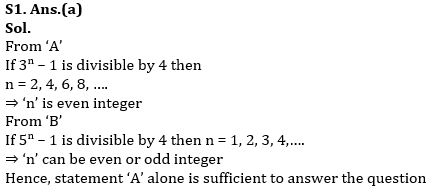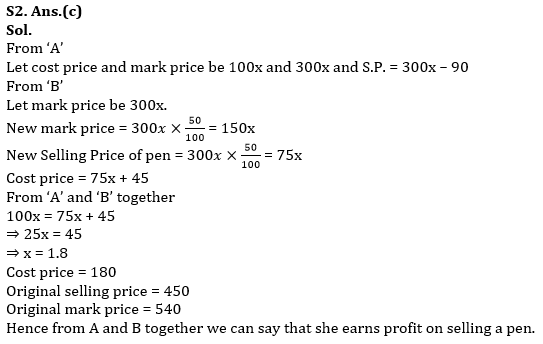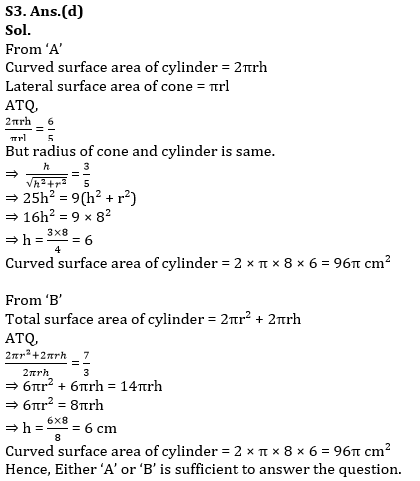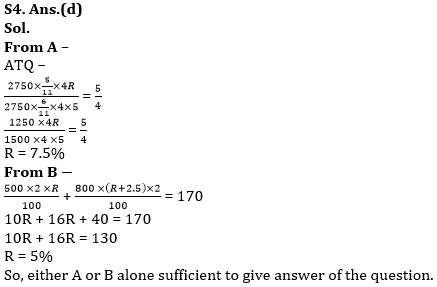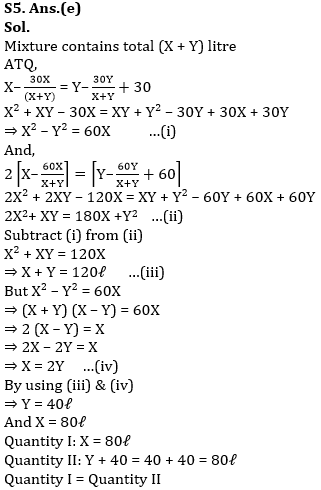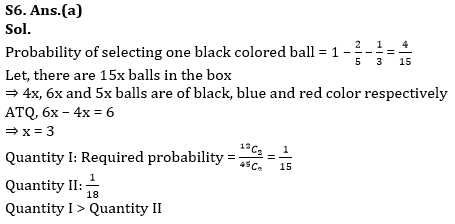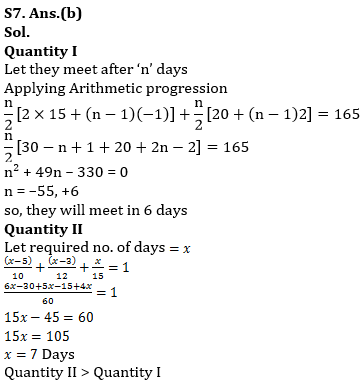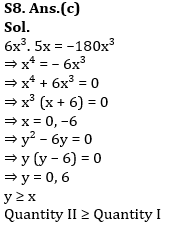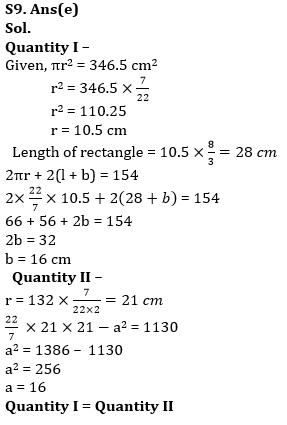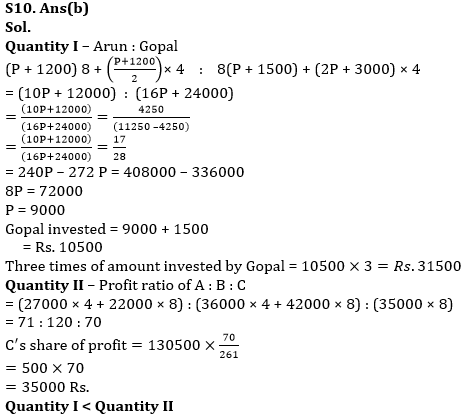×

Thank You, Your details have been submitted we will get back to you.Join India's largest learning destination

What You Will get ?

•Job Alerts
•Daily Quizzes
•Subject-Wise Quizzes
•Current Affairs
•Previous year question papers
•Doubt Solving session

ORJoin India's largest learning destination

What You Will get ?

•Job Alerts
•Daily Quizzes
•Subject-Wise Quizzes
•Current Affairs
•Previous year question papers
•Doubt Solving session

ORJoin India's largest learning destination

What You Will get ?

•Job Alerts
•Daily Quizzes
•Subject-Wise Quizzes
•Current Affairs
•Previous year question papers
•Doubt Solving session

Enter the email address associated with your account, and we'll email you an OTP to verify it's you.Join India's largest learning destination

What You Will get ?

•Job Alerts
•Daily Quizzes
•Subject-Wise Quizzes
•Current Affairs
•Previous year question papers
•Doubt Solving session

Enter OTP

Please enter the OTP sent to
/6

Did not recive OTP?

Resend in 60sJoin India's largest learning destination

What You Will get ?

•Job Alerts
•Daily Quizzes
•Subject-Wise Quizzes
•Current Affairs
•Previous year question papers
•Doubt Solving sessionJoin India's largest learning destination

What You Will get ?

•Job Alerts
•Daily Quizzes
•Subject-Wise Quizzes
•Current Affairs
•Previous year question papers
•Doubt Solving session

Almost there

+91Join India's largest learning destination

What You Will get ?

•Job Alerts
•Daily Quizzes
•Subject-Wise Quizzes
•Current Affairs
•Previous year question papers
•Doubt Solving session

Enter OTP

Please enter the OTP sent to Edit Number

Did not recive OTP?

Resend 60

By skipping this step you will not recieve any free content avalaible on adda247, also you will miss onto notification and job alerts

Are you sure you want to skip this step?

By skipping this step you will not recieve any free content avalaible on adda247, also you will miss onto notification and job alerts

Are you sure you want to skip this step?